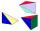Triangular pyramid

Determine the volume and surface area of a regular triangular pyramid having a base edge a=20 cm and a lateral edge b = 35 cm

Result

V =  1848.423 cm3
S =  1179.436 cm2

Solution:Leave us a comment of example and its solution (i.e. if it is still somewhat unclear...):Be the first to comment!To solve this example are needed these knowledge from mathematics:

See also our trigonometric triangle calculator. Pythagorean theorem is the base for the right triangle calculator.

Next similar examples:

1. Triangular pyramidCalculate the volume and surface area of a regular triangular pyramid whose height is equal to the length of the base edges 10 cm.
2. PyramidCuboid ABCDEFGH has dimensions AB 3 cm, BC 4 cm, CG 5 cm. Calculate the volume and surface area of a triangular pyramid ADEC.
3. Pyramid 4sidesCalculate the volume and the surface of a regular quadrangular pyramid when the edge of the base is 4 cm long and the height of the pyramid is 7 cm.Find the volume and surface of a regular quadrilateral pyramid if the bottom edge is 45 cm long and the pyramid height is 7 cm.
5. Hexagonal pyramidBase of the pyramid is a regular hexagon, which can be circumscribed in a circle with a radius of 1 meter. Calculate the volume of a pyramid 2.5 meters high.
6. PyramidThe pyramid has a base rectangle with a = 6cm, b = 8cm. The side edges are the same and their length = 12.5 cm. Calculate the surface of the pyramid.
7. 4s pyramidRegular tetrahedral pyramid has a base edge a=17 and collaterally edge length b=32. What is its height?
8. Cylinder - A&VThe cylinder has a volume 1287. The base has a radius 10. What is the area of surface of the cylinder?
9. TetrahedronCalculate height and volume of a regular tetrahedron whose edge has a length 18 cm.
10. Fit ballWhat is the size of the surface of Gymball (FIT - ball) with a diameter of 65 cm?
11. Volleyball8 girls wants to play volleyball against boys. On the field at one time can be six players per team. How many initial teams of this girls may trainer to choose?
12. Center traverseIt is true that the middle traverse bisects the triangle?
13. Theorem proveWe want to prove the sentence: If the natural number n is divisible by six, then n is divisible by three. From what assumption we started?The double ladder is 8.5m long. It is built so that its lower ends are 3.5 meters apart. How high does the upper end of the ladder reach?The double ladder shoulders should be 3 meters long. What height will the upper top of the ladder reach if the lower ends are 1.8 meters apart?In ▵ ABC, if sin(α)=0.5 and sin(β)=0.6 calculate sin(γ)Find g(1) if g(x) = 3x - x2 Find f(5) if f(x) = x + 1/2